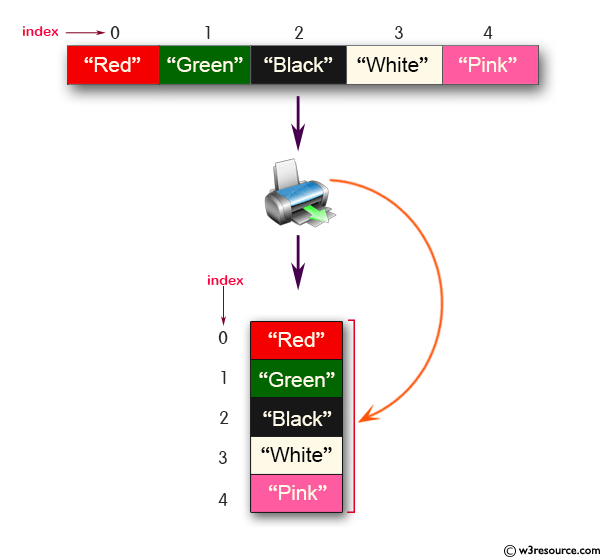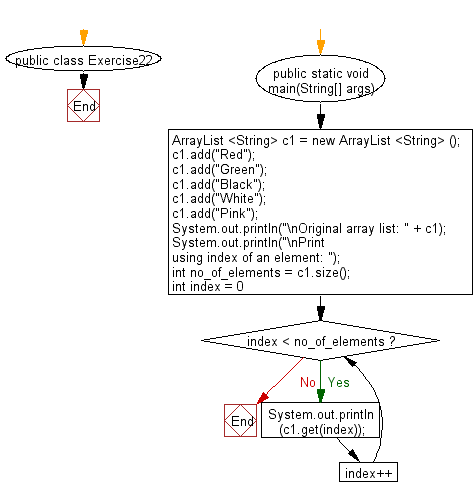﻿ Java: Print ArrayList using the position of the elements# Java Collection, ArrayList Exercises: Print all the elements of a ArrayList using the position of the elements

## Java Collection, ArrayList Exercises: Exercise-22 with Solution

Write a Java program to print all the elements of a ArrayList using the position of the elements.

Pictorial Presentation:Sample Solution:-

Java Code:

``````import java.util.ArrayList;
public class Exercise22 {
public static void main(String[] args) {
ArrayList <String> c1 = new ArrayList <String> ();
System.out.println("\nOriginal array list: " + c1);
System.out.println("\nPrint using index of an element: ");
int no_of_elements = c1.size();
for (int index = 0; index < no_of_elements; index++)
System.out.println(c1.get(index));
}
}
``````

Sample Output:

```Original array list: [Red, Green, Black, White, Pink]

Print using index of an element:
Red
Green
Black
White
Pink
```

Flowchart:Java Code Editor:

What is the difficulty level of this exercise?

Test your Programming skills with w3resource's quiz.

﻿

## Java: Tips of the Day

countOccurrences

Counts the occurrences of a value in an array.

Use Arrays.stream().filter().count() to count total number of values that equals the specified value.

```public static long countOccurrences(int[] numbers, int value) {
return Arrays.stream(numbers)
.filter(number -> number == value)
.count();
}
```

Ref: https://bit.ly/3kCAgLb